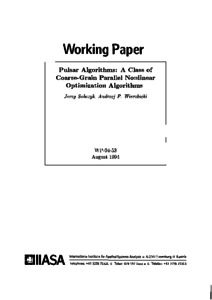# Pulsar Algorithms: A Class of Coarse-Grain Parallel Nonlinear Optimization Algorithms

Sobczyk, J. & Wierzbicki, A.P. (1994). Pulsar Algorithms: A Class of Coarse-Grain Parallel Nonlinear Optimization Algorithms. IIASA Working Paper. IIASA, Laxenburg, Austria: WP-94-053Preview Text WP-94-053.pdf Download (1MB) | Preview

## Abstract

Parallel architectures of modern computers formed of processors with high computing power motivate the search for new approaches to basic computational algorithms. Another motivating force for parallelization of algorithms has been the need to solve very large scale or complex problems. However, the complexity of a mathematical programming problem is not necessarily due to its scale or dimension; thus, we should search also for new parallel computation approaches to problems that might have a moderate size but are difficult for other reasons. One of such approaches might be coarse-grained parallelization based on a parametric imbedding of an algorithm and on an allocation of resulting algorithmic phases and variants to many processors with suitable coordination of data obtained that way. Each processor performs then a phase of the algorithm -- a substantial computational task which mitigates the problems related to data transmission and coordination. The paper presents a class of such coarse-grained parallel algorithms for unconstrained nonlinear optimization, called pulsar algorithms since the approximations of an optimal solution alternatively increase and reduce their spread in subsequent iterations. The main algorithmic phase of an algorithm of this class might be either a directional search or a restricted step determination in a trust region method. This class is exemplified by a modified, parallel Newton-type algorithm and a parallel rank-one variable metric algorithm. In the latter case, a consistent approximation of the inverse of the hessian matrix based on parallel produced data is available at each iteration, while the known deficiencies of a rank-one variable metric are suppressed by a parallel implementation. Additionally, pulsar algorithms might use a parametric imbedding into a family of regularized problems in order to counteract possible effects of ill-conditioning. Such parallel algorithms result not only in an increased speed of solving a problem but also in an increased robustness with respect to various sources of complexity of the problem. Necessary theoretical foundations, outlines of various variants of parallel algorithms and the results of preliminary tests are presented.

Item Type: Monograph (IIASA Working Paper) Methodology of Decision Analysis (MDA) IIASA Import 15 Jan 2016 02:04 27 Aug 2021 17:14 https://pure.iiasa.ac.at/4158View Item